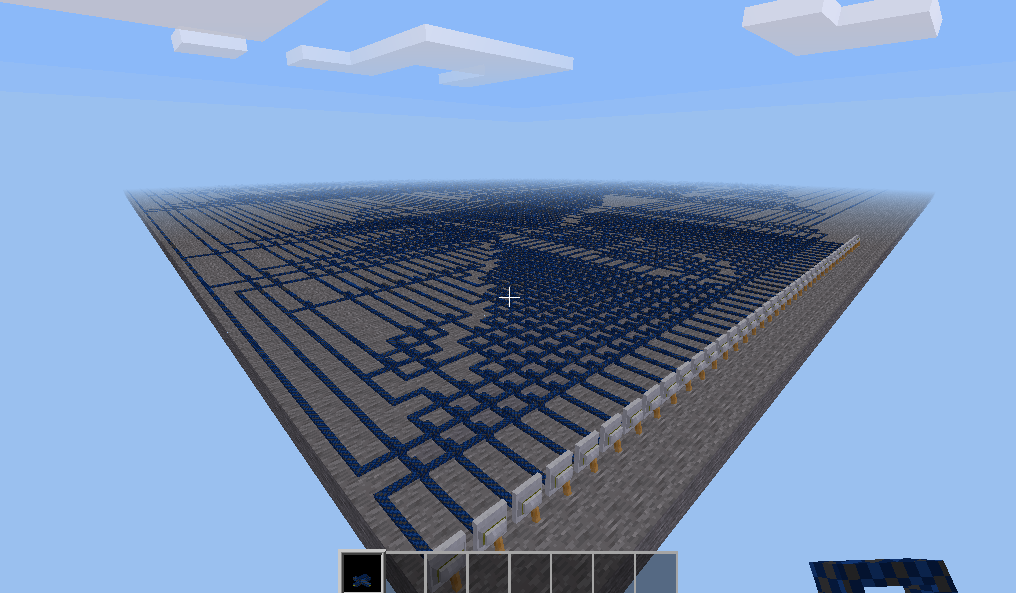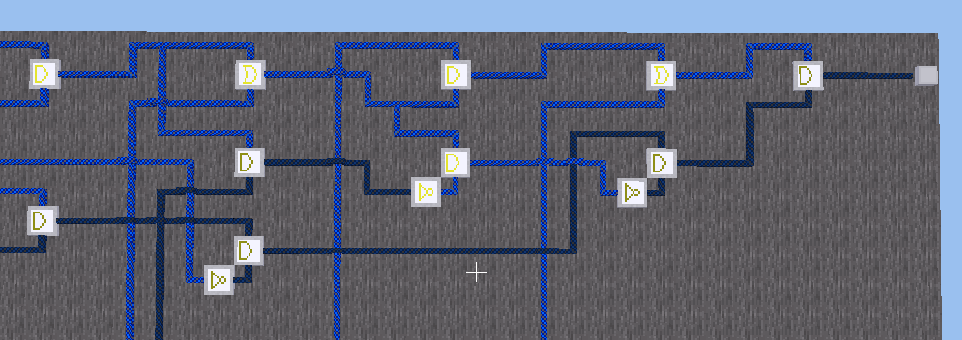# minetestI've stumbled upon this weird minetest map, can you make sense out of it?
Minetest + mesecons required
Non-standard flag format (enter bits as 0 and 1).

5.5 hour

# Behavior

The task is a Minetest (Something like Minecraft) map, and it looks like this:A HUUUUUUUUUUUUGE redstone circuit.

# Solution

## TL;DR

1. Parse the map database.
2. Dump all mesecons blocks' type, orientation, and position.
3. Simplify all interconnections.
4. Solve the boolean expression with z3.

## Minetest map

There's a file named map.sqlite. Clearly, this is what we want.

Open it with sqlite3, and there's only one table inside.

sqlite> .schema
CREATE TABLE blocks (
pos INT PRIMARY KEY,
data BLOB
);


The file format is well documented in here.

In minetest, a voxel (i.e. a basic cube in the game) is called a "node". And 16x16x16 nodes is grouped into one block (i.e. a SQL record).

pos field is a 36-bits compressed integer contains 3 12-bits integers.

data field contains some metadata of the block and a zlib compressed array about each nodes.

I won't put format details here. Just follow its document to write a parser.

There's a lot of blocks only contains air or ignore nodes. Instead of parse whole database, I only parse the mesecons-related blocks with this query:

SELECT pos, data FROM blocks WHERE instr(data, "mesecons") > 0;


You can find the parser from here

Here's a all the blocks I parsed:

air
default:stone

mesecons_insulated:insulated_on
mesecons_insulated:insulated_off

mesecons_extrawires:crossover_01
mesecons_extrawires:crossover_10
mesecons_extrawires:crossover_on
mesecons_extrawires:crossover_off

mesecons_extrawires:corner_on
mesecons_extrawires:corner_off

mesecons_extrawires:tjunction_on
mesecons_extrawires:tjunction_off

mesecons_gates:xor_on
mesecons_gates:xor_off
mesecons_gates:or_on
mesecons_gates:or_off
mesecons_gates:and_on
mesecons_gates:and_off
mesecons_gates:not_on
mesecons_gates:not_off

mesecons_lamp:lamp_off
mesecons_walllever:wall_lever_off


And the lamp is in (1, 2, 1938), which looks like:Each node has two parameters, after comparing to the rendered result in the game. I figure out one of the parameters is its orientation.

When orientation is zero, all gates and wire points to the east (i.e. +x direction), corner looks like ┐, and tjunction looks like T.

Orientation 1 rotates 90° clock-wise, 2 rotates 180° clock-wise, 3 rotates 270° clock-wise.

Now, we are ready to reconstruct the circuit.

## Simplify the circuit

In each nodes, there are four connection pins:

• (x - 0.5, y)
• (x + 0.5, y)
• (x, y - 0.5)
• (x, y + 0.5)

And I do the following things to reconstruct the circuit

1. Create an edge list for each pins according to the node type and orientation.
2. Simplify the edges by finding connected components.
3. Remove those unconnected pins
4. Sort the gates topologically. (The circuit in this task is a DAG)

Now it looks like:

  type       out    inputs
('lever_0',  0,    [          ]),
('lever_1',  1,    [          ]),
('lever_2',  2,    [          ]),
('lever_3',  3,    [          ]),
...
('lever_37', 37,   [          ]),
('lever_38', 38,   [          ]),
('lever_39', 39,   [          ]),
('not',      40,   [34        ]),
('not',      41,   [31        ]),
('not',      42,   [30        ]),
...
('and',      3356, [3348, 3355]),
('and',      3357, [1274, 3353]),
('or',       3358, [3357, 1125]),
('and',      3359, [3358, 3356]),
('lamp',     None, [3359      ])


While I was trying to understand the circuit with graphviz, my teammate found the solution with z3 solver.

He converted the constraint above to the following code using some regex:

from z3 import *

v_0 = Bool('lever_0')
v_1 = Bool('lever_1')
v_2 = Bool('lever_2')
...
v_37 = Bool('lever_37')
v_38 = Bool('lever_38')
v_39 = Bool('lever_39')
v_40 = Not( v_34 )
v_41 = Not( v_31 )
v_42 = Not( v_30 )
...
v_3356 = And( v_3348, v_3355 )
v_3357 = And( v_1274, v_3353 )
v_3358 = Or( v_3357, v_1125 )
v_3359 = And( v_3358, v_3356 )

print("Solving")
print(solve(v_3359, True))


Run it, and you'll get the flag.